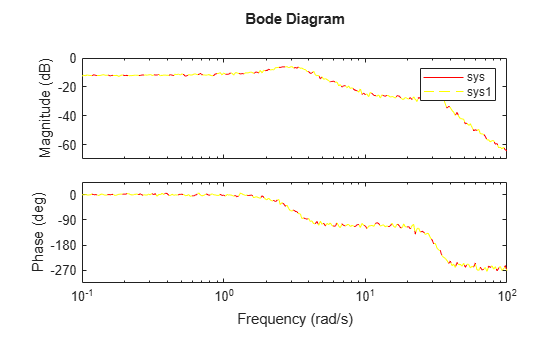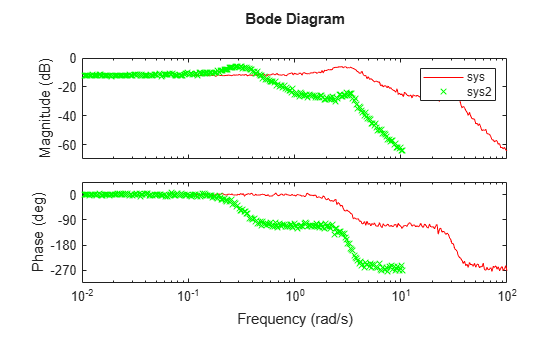# chgFreqUnit

Change frequency units of frequency-response data model

## Syntax

```sys_new = chgFreqUnit(sys,newfrequnits) ```

## Description

```sys_new = chgFreqUnit(sys,newfrequnits)``` changes units of the frequency points in `sys` to `newfrequnits`. Both `Frequency` and `FrequencyUnit` properties of `sys` adjust so that the frequency responses of `sys` and `sys_new` match.

## Input Arguments

 `sys` Frequency-response data (`frd` (Control System Toolbox), `idfrd`, or `genfrd` (Control System Toolbox)) model. `newfrequnits` New units of frequency points, specified as one of the following values: `'rad/TimeUnit'``'cycles/TimeUnit'``'rad/s'``'Hz'``'kHz'``'MHz'``'GHz'``'rpm'` `rad/TimeUnit` and `cycles/TimeUnit` express frequency units relative to the system time units specified in the `TimeUnit` property. Default: `'rad/TimeUnit'`

## Output Arguments

 `sys_new` Frequency-response data model of the same type as `sys` with new units of frequency points. The frequency response of `sys_new` is same as `sys`.

## Examples

collapse all

Create a frequency-response data model.

```load('AnalyzerData'); sys = frd(resp,freq);```

The data file `AnalyzerData` has column vectors `freq` and `resp`. These vectors contain 256 test frequencies and corresponding complex-valued frequency response points, respectively. The default frequency units of `sys` is `rad/TimeUnit`, where `TimeUnit` is the system time units.

Change the frequency units.

`sys1 = chgFreqUnit(sys,'rpm');`

The `FrequencyUnit` property of `sys1` is `rpm`.

Compare the Bode responses of `sys` and `sys1`.

```bodeplot(sys,'r',sys1,'y--'); legend('sys','sys1')```The magnitude and phase of `sys` and `sys1` match because `chgFreqUnit` command changes the units of frequency points in `sys` without modifying system behavior.

Change the `FrequencyUnit` property of `sys` to compare the Bode response with the original system.

```sys2 = sys; sys2.FrequencyUnit = 'rpm'; bodeplot(sys,'r',sys2,'gx'); legend('sys','sys2');```Changing the `FrequencyUnit` property changes the system behavior. Therefore, the Bode responses of `sys` and `sys2` do not match. For example, the original corner frequency at about 2 rad/s changes to approximately 2 rpm (or 0.2 rad/s).

## Tips

• Use `chgFreqUnit` to change the units of frequency points without modifying system behavior.

## Version History

Introduced in R2012a